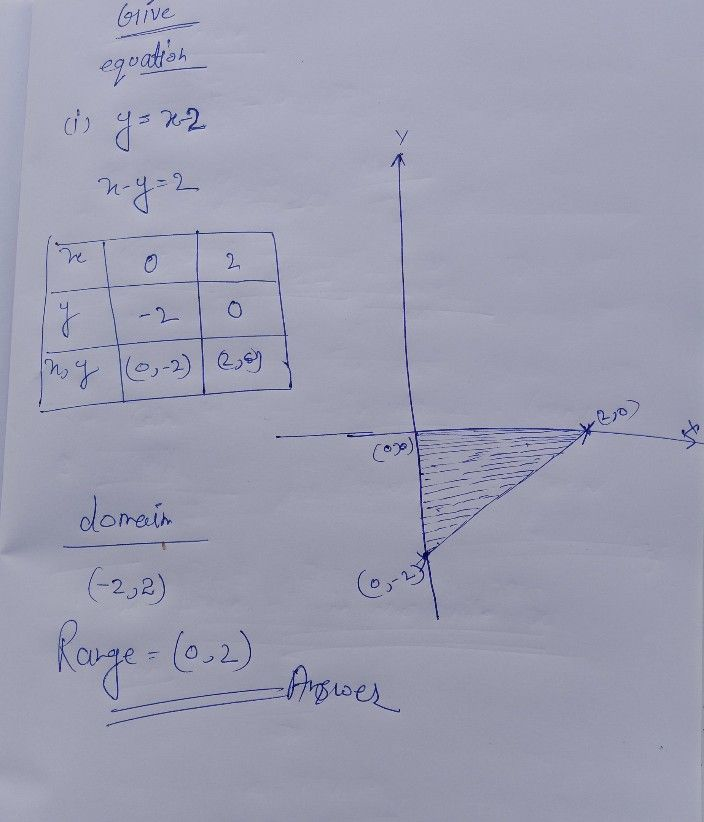Symbol
ProblemAdditional Activities then find Graph the following logarithmic functions using an online graphing calculator its domain and range. $1.$ $y=\left(x-2\right)$ $V-$ $5$ $y=\left(x+1\right)$ $9.y=x+2$ $y=\left(x+3\right)$ $6.y=x-2$ $10.y=x+1$ $y=\left(x-1\right)$ $7.y=x+3$ $11$
10th-13th grade
Other
Search count: 107
Question content
help please
SolutionQanda teacher - henryseeStudent
can u answer the the other pls
sir
thank you very much